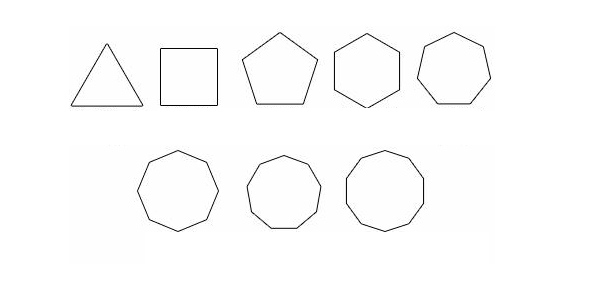# Polygons And Circles

9 Questions | Total Attempts: 241SettingsAs a child, there’s a point in life where shapes and what we now have come to refer as “geometry” are the most fascinating things in the world. Do you really know everything about these 2-D polygons and circles, however?

Related Topics
• 1.
What is a perimeter?
• A.

The line around a polygon.

• B.

The area in a polygon.

• C.

A square.

• 2.
What is the calculation for a the cicumference of a circle?
• A.

3.14 x r

• B.

3.14 x d

• C.

3.14 x d squared

• 3.
Find the area of a triange whos sides whose base is 13 mm, whose height is 5 mm, and where side 1 = 7 mm, side 2 = 11 mm and side 3 = 13 mm?
• A.

18 mm

• B.

65 square mm

• C.

65 mm

• 4.
Find the perimeter of a triangle whose sides are base is 13 mm, whose height is 5 mm, and where side 1 = 7 mm, side 2 = 11 mm and base = 13 mm?
• A.

15.5 mm

• B.

31 mm

• C.

29 mm

• 5.
Find the area of a parallelogram whos sides are 14 m and 20 m and whose base = 10m?
• A.

140 sq m

• B.

200 sq m

• C.

280 sq m

• 6.
Find the perimeter of a parallelogram whos sides are 14 m and 20 m and whose base = 10m?
• A.

34 m

• B.

56 m

• C.

68 m

• 7.
Find the area of a circle whose diameter is 16 m?
• A.

200.96 sq m

• B.

156 sq m

• C.

176.22 sq m

• 8.
Find the perimeter of a circle whose diameter is 16 m?
• A.

50.24 inches

• B.

50.24 m

• C.

50.24 sq m

• 9.
What is the formula for calculating the area and perimeter of a square?
• A.

B x h, l x w

• B.

2l + 2w, l x w

• C.

S squared and 4S